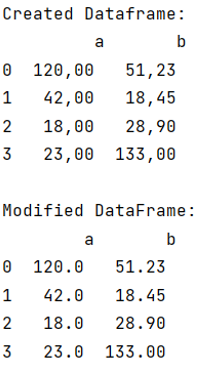# Python Pandas: Convert commas decimal separators to dots within a Dataframe

Given a Pandas DataFrame, we have to convert commas decimal separators to dots within a Dataframe.
Submitted by Pranit Sharma, on July 28, 2022

Pandas is a special tool that allows us to perform complex manipulations of data effectively and efficiently. Inside pandas, we mostly deal with a dataset in the form of DataFrame. DataFrames are 2-dimensional data structures in pandas. DataFrames consist of rows, columns, and data.

Sometimes we have a faulty data value in our data set or there are some values that we want in different data types, in that case, we need to either change the data type of the value or replace all these values with some particular value.

But sometimes, we need to do both tasks to achieve our optimum goal. For this purpose, we will use str.replace() method.

## df.str.replace() Method

This method is used when we need to replace some string with another string, it returns the updated complete string as a result.

Syntax:

```df['col'].replace('value_to_be_replaced','alternate_value',regex=True)
```

Let us understand with the help of an example,

## Python code to convert commas decimal separators to dots within a Dataframe

```# Importing Pandas package
import pandas as pd

# Creating a Dictionary
d = {
'a': ['120,00', '42,00', '18,00', '23,00'],
'b': ['51,23', '18,45', '28,90', '133,00']
}

# Creating a dataframe
df = pd.DataFrame(d)

# Display Original dataframe
print("Created Dataframe:\n",df,"\n")

# Replacing , with .
df['a'] = df['a'].replace(',','.',regex=True)
df['b'] = df['b'].replace(',','.',regex=True)

# Converting the data type
df['a'] = df['a'].astype(float)
df['b'] = df['b'].astype(float)

# Display modified DataFrame
print("Modified DataFrame:\n",df)
```

Output:Languages: » C » C++ » C++ STL » Java » Data Structure » C#.Net » Android » Kotlin » SQL
Web Technologies: » PHP » Python » JavaScript » CSS » Ajax » Node.js » Web programming/HTML
Solved programs: » C » C++ » DS » Java » C#
Aptitude que. & ans.: » C » C++ » Java » DBMS
Interview que. & ans.: » C » Embedded C » Java » SEO » HR
CS Subjects: » CS Basics » O.S. » Networks » DBMS » Embedded Systems » Cloud Computing
» Machine learning » CS Organizations » Linux » DOS
More: » Articles » Puzzles » News/Updates